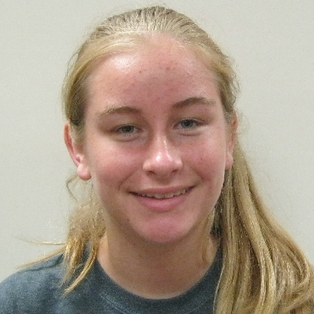# Combinatorics Seminars

Upcoming Combinatorics Seminars
DMS Discrete Mathematics Seminar
Oct 03, 2023 02:00 PM
354 Parker HallSpeaker: Pete Johnson

Title: Some curious results concerning certain geometrically defined hypergraphs

Past Combinatorics Seminars
DMS Discrete Mathematics Seminar
Sep 28, 2023 02:00 PM
354 Parker HallSpeaker: Chris Wells

DMS Discrete Mathematics Seminar
Sep 14, 2023 02:00 PM
354 Parker Hall

Speaker: Songling Shan (Auburn University)

Title: Toughness and the existence of $$k$$-factors in hypergraphs

Abstract: In 1973, Chvátal introduced the concept of graph toughness and initiated the study of hamiltonian cycles under toughness conditions. Many classic results related to graphs toughness were later on proved including the famous result by Enomoto,  Jackson, Keterinis, and Saito from 1985, that for every integer $$k\ge 1$$, a graph $$G$$ has a  $$k$$-factor if  $$G$$ is $$k$$-tough, $$k |V(G)|$$ is even, and $$|V(G)|\ge k+1$$.

As a natural analogy of the concepts of cycles in graphs,  Berge cycles in hypergraphs have recently attracted more attentions of researchers and their existence was mostly studied under degree conditions.  In this paper, we study Berge $$k$$-factors, an analogy of $$k$$-factors,  in hypergraphs, and extend the classic result of Enomoto,  Jackson, Keterinis, and Saito to hypergraphs.  The proof is more complicated than the proof for the graph case as we do not require the hypergraph to be uniform.

DMS Discrete Mathematics
Sep 07, 2023 02:00 PM
ZOOMShannon Ogden (Victoria U)

Title: The Rainbow Saturation Number is Linear

Abstract: An edge-coloured graph is said to be rainbow if every edge in the graph has a distinct colour. Given a graph H, an edge-coloured graph G is H-rainbow saturated if it does not contain a rainbow copy of H, but the addition of any non-edge, in any colour, creates a rainbow copy of H. The rainbow saturation number, denote by rsat(n,H), is the minimum number of edges in an H-rainbow saturated edge-coloured graph on n vertices. In this talk, we will show that, for any non-empty graph H, the rainbow saturation number is linear in n, thus proving a conjecture of Girão, Lewis, and Popielarz. Based on work with Natalie Behague, Tom Johnston, Shoham Letzter, and Natasha Morrison.

DMS Combinatorics Seminar
Apr 25, 2023 02:00 PM
ZOOMSpeaker: Ashna Wright (University of Victoria)

Title: Improved bounds for cross-Sperner systems

DMS Combinatorics Seminar
Apr 20, 2023 02:00 PM
ZOOMSpeaker:  Tash Morrison, University of Victoria (Canada)

TBA

DMS Combinatorics Seminar
Apr 13, 2023 02:00 PM
328 Parker Hall

Speaker: Derrick DeMars, Auburn

Title: Forbidding Monochromatic and Rainbow Cycles and Families of Cycles

DMS Combinatorics Seminar
Apr 11, 2023 02:00 PM
328 Parker HallSpeaker: Stacie Baumann

Title: Embedding and Coloring Designs

Abstract: This presentation focuses on the two problems contained in my dissertation.

The main topic of the presentation is the first problem that concerns completing partial latin squares with prescribed diagonals. Necessary and sufficient numerical conditions are known for the embedding of an incomplete latin square $$L$$ of order $$n$$ into a latin square $$T$$ of order $$t ≥ 2n + 1$$ in which each symbol is prescribed to occur in a given number of cells on the diagonal of $$T$$ outside of $$L$$. This includes the classic case where $$T$$ is required to be idempotent. If $$t < 2n$$ then no such numerical sufficient conditions exist since it is known that the arrangement of symbols within the given incomplete latin square can determine the embeddability. All known examples where the arrangement is a factor share the common feature that one symbol is prescribed to appear exactly once in the diagonal of $$T$$ outside of $$L$$. We show if the prescribed diagonal contains a symbol required to appear exactly once on the diagonal and $$t ≤ 2n$$, then there always exists a incomplete latin square satisfying the known numerical necessary conditions that is non-embeddable. Also, we solve a conjecture made over 30 years ago stating it is only this feature that prevents numerical conditions sufficing for all $$t ≥ n$$. Thus providing necessary and sufficient numerical conditions for the embedding of an incomplete latin square $$L$$ of order $$n$$ into a latin square $$T$$ of order $$t$$ for all $$t ≥ n$$ in which the diagonal of $$T$$ outside of $$L$$ is prescribed in the case where no symbol is required to appear exactly once in the diagonal of $$T$$ outside of $$L$$.

The presentation then briefly summarizes the second problem that concerns (not necessarily proper) $$s$$-edge-colorings of $$K_{v}$$ in which, for all  $$u ∈ V(K_{v})$$, the edges incident with $$u$$ are colored using exactly $$p$$ colors. In the spirit of proper edge-colorings, such $$(s, p)$$-edge-colorings are required to be equitable: the edges at each vertex are shared evenly among $$p$$ colors. Results and future directions are stated.

DMS Combinatorics Seminar
Apr 06, 2023 02:00 PM
328 Parker HallSpeaker: Isabel Harris

Title: General Results on $$k$$-Rainbow Avoiding Subgraphs

Abstract: A simple graph with $$e = E(G)$$ avoids a $$k$$-rainbow coloring if any color appears on at least $$k+1$$ edges of $$G$$. For $$k ∈ P, ARk(G, n)$$ is the maximum number of colors in an edge coloring of $$Kn$$ so that in every copy of $$G$$, some color occurs on at least $$k+1$$ edges. $$G$$ is $$ARk$$-bounded if $$ARk(G,n) ≤ c$$ for some $$c ∈ P$$ and all $$n$$ sufficiently large.  In this talk we will discuss some results on finding $$ARk$$-bounded graphs for any $$k$$.

DMS Combinatorics Seminar
Apr 04, 2023 02:00 PM
328 Parker HallSpeaker: Elizabeth Schloss

DMS Combinatorics Seminar
Mar 30, 2023 09:00 AM
ZOOMSpeaker: Debsoumya Chakraborti, Institute for Basic Science (IBS), South Korea

Title: Rainbow extremal problem for color-critical graphs

Abstract: Given $$k$$ graphs $$G_1,\dots, G_k$$ over a common vertex set of size $$n$$, what conditions on $$G_i$$ ensure a rainbow copy of $$H$$, i.e., a copy of $$H$$ with at most one edge from each $$G_i$$? We study the problem of maximizing $$\sum_{i} e(G_i)$$ without a rainbow copy of $$H$$, where $$e(G_i)$$ denotes the number of edges in the graph $$G_i$$. We discuss some results and open questions when $$H$$ is a complete or a color-critical graph. We also discuss our recent progress on this problem to a conjecture of Keevash, Saks, Sudakov, and Verstraete.

This talk will be based on joint work with Kim, Lee, Liu, and Seo.